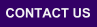Mplus
Friday
January 15, 2021Mplus Mplus at a GlanceGeneral DescriptionMplus Programs Pricing Version History System Requirements Platforms FAQ
 Analyses/ResearchMplus Examples Papers References
 Search

Chapter 10: Missing data

Table 10.1 (Part 1), pp. 441-442: Sample statistics estimated by multiple imputation followed by ML 10.1 (Part 1) 10.1 (Part 1) .inp Data are unavailable
Table 10.1 (Part 2), pp. 441-442: Sample statistics estimated by Bayes using an unrestricted model 10.1 (Part 2) 10.1 (Part 2) .inp Data are unavailable
Table 10.4, p. 451: Step 1 Monte Carlo simulation assuming MAR: Generating the data 10.4 10.4 .inp N/A
Table 10.5, pp. 452-453: Step 2 external Monte Carlo simulation assuming MAR: Analyzing the data including the missing data indicator 10.5 10.5 .inp Generated by 10.4
Table 10.7, p. 454: Step 2 external Monte Carlo simulation assuming MAR: Analyzing the data excluding the missing data indicator 10.7 10.7 .inp Generated by 10.4
Table 10.9, p. 455: Step 2 external Monte Carlo simulation assuming MAR: Analyzing the data excluding the missing data indicator and using listwise 10.9 10.9 .inp Generated by 10.4
Table 10.11, p.459: Monte Carlo simulation with missingness due to a missing data correlate z 10.11 10.11 .inp N/A
Table 10.12, p.461: Step 2 external Monte Carlo simulation including the missing data correlate z as a covariate 10.12 10.12 .inp Generated by 10.11
Table 10.13, p. 461: Step 2 external Monte Carlo simulation excluding the missing data correlate z 10.13 10.13 .inp Generated by 10.11
Table 10.14, pp. 462-463: Step 2 external Monte Carlo simulation excluding the missing data correlate z and using listwise 10.14 10.14 .inp Generated by 10.11
Table 10.15, pp. 462-463: Step 2 external Monte Carlo simulation using AUXILIARY = z(M) 10.15 10.15 .inp Generated by 10.4
Table 10.17, p. 467: Monte Carlo simulation under NMAR with missingness predicted by a variable with missing data: Generating the data 10.17 10.17 .inp N/A
Table 10.18, p. 468: Step 2 external Monte Carlo simulation under NMAR: Analyzing the data including the missing data indicator using selection modeling 10.18 10.18 .inp Generated by 10.17
Table 10.19, p. 468: Step 2 external Monte Carlo simulation under NMAR: ML assuming MAR 10.19 10.19 .inp Generated by 10.17
Table 10.20, p. 469: Step 2 external Monte Carlo simulation under NMAR: Listwise 10.20 10.20 .inp Generated by 10.17
Table 10.22, p. 471: Step 2 external Monte Carlo simulation under MAR: Selection modeling 10.22 10.22 .inp Generated by 10.4
Table 10.24, p. 473: Aggression mediation modeling with multiple imputation of agg1 (approach 9 in Table 10.25) 10.24 10.24 .inp Data are unavailable
Table 10.25 (Approach 1), p. 474: Listwise (n=250) 10.25 (Approach 1) 10.25 (Approach 1) .inp Data are unavailable
Table 10.25 (Approach 2), p. 474: ML assuming MAR (n=392) 10.25 (Approach 2) 10.25 (Approach 2) .inp Data are unavailable
Table 10.25 (Approach 3), p. 474: ML assuming MAR with black as missing data correlate (n=392) 10.25 (Approach 3) 10.25 (Approach 3) .inp Data are unavailable
Table 10.25 (Approach 4), p. 474: ML on multiple imputation of agg5 (n=392) 10.25 (Approach 4) 10.25 (Approach 4) .inp Data are unavailable
Table 10.25 (Approach 5), p. 474: Bayes (n=392) 10.25 (Approach 5) 10.25 (Approach 5) .inp Data are unavailable
Table 10.25 (Approach 6), p. 474: Selection modeling, missing regressed on agg5 (n=392) 10.25 (Approach 6) 10.25 (Approach 6) .inp Data are unavailable
Table 10.25 (Approach 7), p. 474: ML assuming MAR (n=441) 10.25 (Approach 7) 10.25 (Approach 7) .inp Data are unavailable
Table 10.25 (Approach 8), p. 474: ML assuming MAR with black as missing data correlate (n=441) 10.25 (Approach 8) 10.25 (Approach 8) .inp Data are unavailable
Table 10.25 (Approach 9), p. 474: ML on multiple imputation of agg1 (n=441) 10.25 (Approach 9) 10.25 (Approach 9) .inp Data are unavailable
Table 10.25 (Approach 10), p. 474: Bayes (n=441) 10.25 (Approach 10) 10.25 (Approach 10) .inp Data are unavailable
Table 10.25 (Approach 11), p. 474: Selection modeling, missing regressed on agg5 (n=441) 10.25 (Approach 11) 10.25 (Approach 11) .inp Data are unavailable
Table 10.25 (Approach 12), p. 474: ML assuming MAR (n=441) 10.25 (Approach 12) 10.25 (Approach 12) .inp Data are unavailable
Table 10.25 (Approach 13), p. 474: ML on multiple imputation of agg1 (n=441) 10.25 (Approach 13) 10.25 (Approach 13) .inp Data are unavailable
Table 10.25 (Approach 14), p. 474: Bayes (n=441) 10.25 (Approach 14) 10.25 (Approach 14_ .inp Data are unavailable
Table 10.25 (Approach 15), p. 474: Selection modeling, missing regressed on agg5 (n=441) 10.25 (Approach 15) 10.25 (Approach 15) .inp Data are unavailable
Table 10.25 (Approach 16), p. 474: Selection modeling, missing regressed on agg5 and black (n=441) 10.25 (Approach 16) 10.25 (Approach 16) .inp Data are unavailable
Table 10.26, p. 477: Step 1 Monte Carlo simulation generating MAR missing data, n=200, 40% missing 10.26 10.26 .inp N/A
Table 10.27, p. 478: Step 2 external Monte Carlo simulation: MLR assuming normality 10.27 10.27 .inp Generated by 10.26
Table 10.28, p. 479: Step 2 external Monte Carlo simulation: Bayes assuming normality 10.28 10.28 .inp Generated by 10.26
Table 10.29, p. 479: Step 2 external Monte Carlo simulation: Bayes treating binary xs as binary 10.29 10.29 .inp Generated by 10.26
Table 10.30, p. 481: Step 1 Monte Carlo simulation generating NMAR missing data, n=200, 40% missing 10.30 10.30 .inp N/A
Table 10.31, p. 481: Step 2 external Monte Carlo simulation under NMAR: MLR assuming normality 10.31 10.31 .inp Generated by 10.30
Table 10.32, p. 482: Step 2 external Monte Carlo simulation under NMAR: Bayes assuming normality 10.32 10.32 .inp Generated by 10.30
Table 10.33, p. 482: Step 2 external Monte Carlo simulation under NMAR: Bayes treating binary xs as binary 10.33 10.33 .inp Generated by 10.30